### Home > CC2 > Chapter Ch8 > Lesson 8.1.1 > Problem8-13

8-13.
1. Mrs. Ferguson, your school librarian, asks you to conduct a survey of how many books students read during the year. You get the following results: 12, 24, 10, 32, 12, 4, 35, 10, 8, 12, 15, 20, 18, 25, 21, and 9. Homework Help ✎

1. Use the data to create a histogram. Use a bin width of 10 books. (Remember, if a value falls on the line, place it in the upper bin.)

2. Is the mean or median a better measure of the center? Find the value of whichever is more appropriate.

3. Make a box plot of the data.

4. Describe the center, shape, spread, and outliers of the distribution.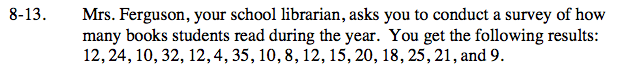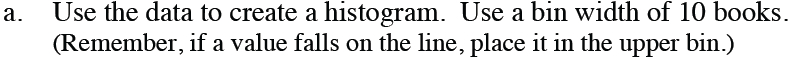Try putting the data in order to make creating the histogram easier.

What are the two axes of the histogram? How can you arrange the data to fit these two axes?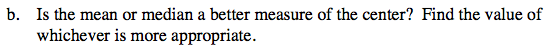Remember that the mean is the average of the data, and the median is the value in the middle of all the data.

Because the data is almost symmetric with no outliers, either the mean or median is appropriate.
Mean = 16.69 books
Median = 13.5 books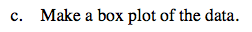Think of the parts of a box plot. How can you use the data to create one?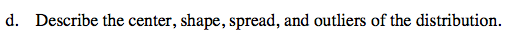The typical student reads about 13.5 books, and the distribution is almost symmetrical and single-peaked.
The IQR is 12.5 so there is a lot of variability in the number of books read, but no apparent outliers.